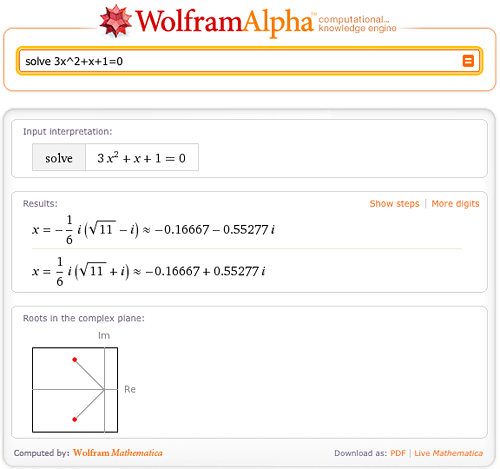TAG: Integers
January 14, 2010–

Need a tutor for solving equations? Solving equations is just one of hundreds of mathematical tasks that can be done using Wolfram|Alpha. Wolfram|Alpha can solve equations from middle school level all the way through college level and beyond. So next time you are stumped on an equation, consult Wolfram|Alpha for a little help.

Let’s start with the simpler stuff. Wolfram|Alpha can easily solve linear and quadratic equations, and even allows you to view a step-by-step solution of each problem.What if the roots of the equation are complex? No worries; Wolfram|Alpha has no trouble solving equations over the complex plane.Wolfram|Alpha can also solve cubic and quartic equations in terms of radicals.Of course, some solutions are too large or cannot be represented in terms of radicals; Wolfram|Alpha will then return numerical solutions with a “More digits” button. More »

June 8, 2009–

There’s new data flowing into Wolfram|Alpha every second. And we’re always working very hard to develop the core code and data for the system. In fact, internally, we have a complete new version of the system that’s built every day. But before we release this version for general use, we do extensive validation and testing.

In addition to real-time data updates, we’ve made a few changes to Wolfram|Alpha since its launch three weeks ago. But today, as one step in our ongoing, long-term development process, we’ve just made live the first broad updates to the core code and data of Wolfram|Alpha. More »